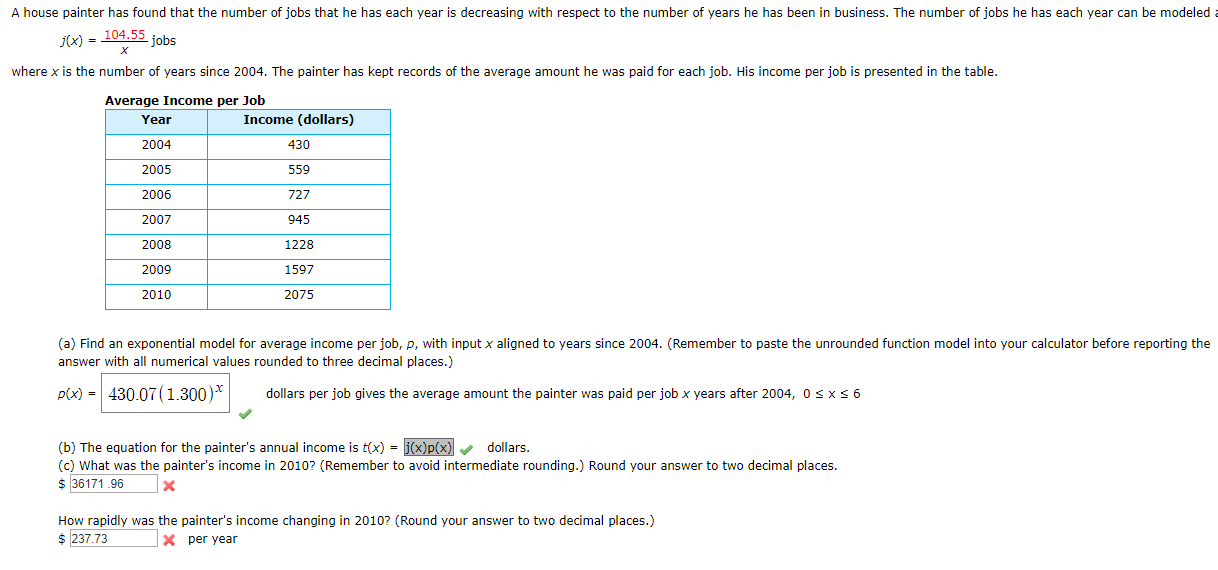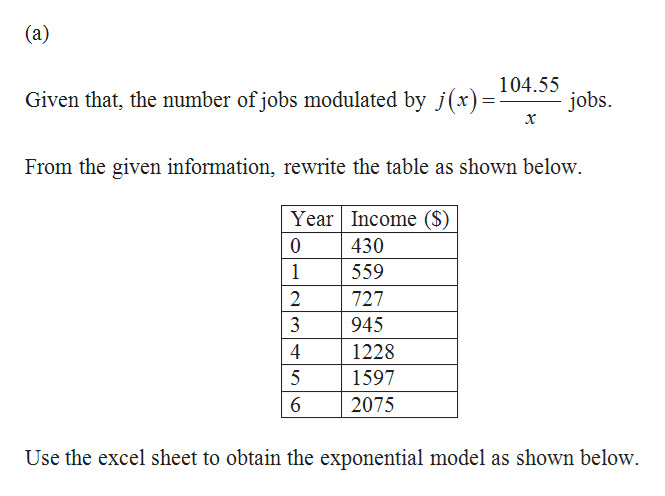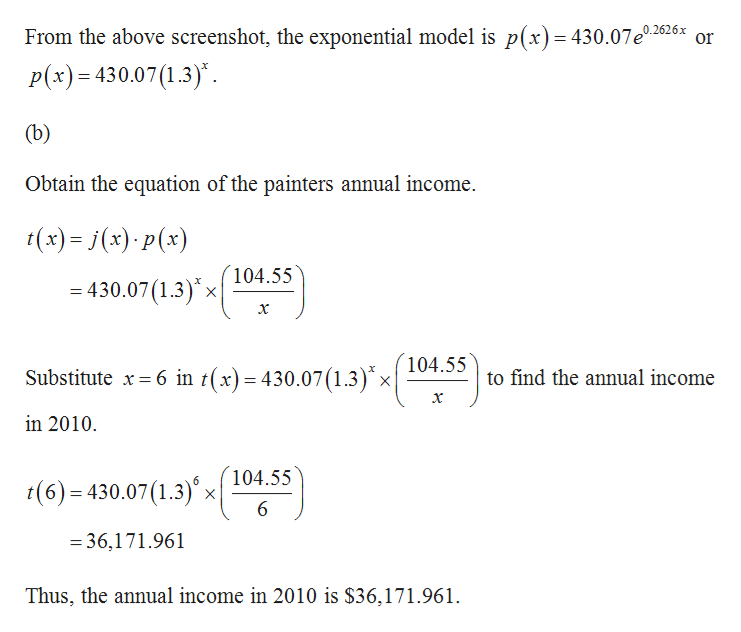# A house painter has found that the number of jobs that he has each year is decreasing with respect to the number of years he has been in business. The number of jobs he has each year can be modeledj(x) = 104.55 jobsXwhere x is the number of years since 2004. The painter has kept records of the average amount he was paid for each job. His income per job is presented in the table.Average Income per JobIncome (dollars)Year2004430200555920067272007945200812282009159720102075(a) Find an exponential model for average income per job, p, with input x aligned to years since 2004. (Remember to paste the unrounded function model into your calculator before reporting theanswer with all numerical values rounded to three decimal places.)p(x) 430.07(1.300)*dollars per job gives the average amount the painter was paid per job x years after 2004, 0 s x s 6(b) The equation for the painter's annual income is tx) = j(x)p(x)dollars.(c) What was the painter's income in 2010? (Remember to avoid intermediate rounding.) Round your answer to two decimal places.36171.96How rapidly was the painter's income changing in 2010? (Round your answer to two decimal places.)\$237.73Xper year

Question
50 viewshelp_outlineImage TranscriptioncloseA house painter has found that the number of jobs that he has each year is decreasing with respect to the number of years he has been in business. The number of jobs he has each year can be modeled j(x) = 104.55 jobs X where x is the number of years since 2004. The painter has kept records of the average amount he was paid for each job. His income per job is presented in the table. Average Income per Job Income (dollars) Year 2004 430 2005 559 2006 727 2007 945 2008 1228 2009 1597 2010 2075 (a) Find an exponential model for average income per job, p, with input x aligned to years since 2004. (Remember to paste the unrounded function model into your calculator before reporting the answer with all numerical values rounded to three decimal places.) p(x) 430.07(1.300)* dollars per job gives the average amount the painter was paid per job x years after 2004, 0 s x s 6 (b) The equation for the painter's annual income is tx) = j(x)p(x) dollars. (c) What was the painter's income in 2010? (Remember to avoid intermediate rounding.) Round your answer to two decimal places. 36171.96 How rapidly was the painter's income changing in 2010? (Round your answer to two decimal places.) \$237.73 Xper year fullscreen
check_circle

Step 1help_outlineImage Transcriptionclose(a) 104.55 Given that, the number of jobs modulated by j(x) = jobs х From the given information, rewrite the table as shown below Year Income (\$) 430 0 1 559 2 727 3 945 4 1228 5 1597 2075 Use the excel sheet to obtain the exponential model as shown below. fullscreen
Step 2
Step 3help_outlineImage TranscriptioncloseFrom the above screenshot, the exponential model is p(x)= 430.07e2626x or P(x)= 430.07 1.3) (b) Obtain the equation of the painters annual income. (x)j(x) p(x) 104.55 = 430.07 (1.3)x| х 104.55 Substitute x6 in t(x)= 430.07 (1.3)* x to find the annual income х in 2010 104.55 t(6)- 430.07(1.3)'x 36,171.961 Thus, the annual income in 2010 is \$36,171.961. fullscreen

### Want to see the full answer?

See Solution

#### Want to see this answer and more?

Solutions are written by subject experts who are available 24/7. Questions are typically answered within 1 hour.*

See Solution
*Response times may vary by subject and question.
Tagged in

### Calculus